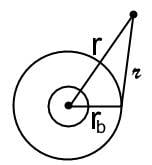# Integrating with Law of Cos

cbirk
I hope I am posting this in the correct area. This is not specificly a homework question, but something that keeps stumping me on numerous Electrostatic problems.

When attempting to Intigrate for finding the electric field or potential, I frequently end up with an integral over the form ∫∫∫ $r^{2}$ / ( $r^{2}$ + $b^{2}$ - 2rb cosθ) dr sinθ dθ dΦ

I was wondering if there is some sort of method the integrate this that I seem to be missing. A basic U sub doesn't work, and I can't get Integration by parts to do anything but make it worse. I have never been very good at remembering the trig tricks to integration so I was really hoping someone could help me out here. I am lost, and this is costing me lots of points on homeworks and tests.

Also after being stumped for days, I am pretty sure that the way the law of cosines is layed out... that cosθ is equivelent to Adjasent / Hyp = b/r which would simplify the entire denominator to a simple $r^{2}$ - $b^{2}$